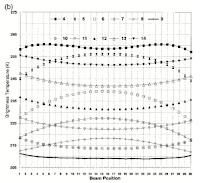## Wednesday, February 3, 2010

### Some Useful Climate Code

I'd hoped to post a C++ AMSU HDF-EOS Level 1B (man, that's a mouthful) reader today. But I'm having trouble locating some of the libraries the downloaded sample code needs. I may have to re-write the reader myself.

So in the meantime I figured I'd post a few useful utilities I've come across while writing this series of posts.

Previous Posts In This Series

Compute Radiance From Frequency And Temperature
This code converts a reading in degrees Kelvin and a frequency value to a radiance value. Radiance is given in units of milliwatts/m2 cm-1. The original C code can be found in the file read_chan_props.c in the C Readers Code provided by NASA. The example here uses pseudocode.

```Function ComputeRadiance    Parameter Temperature Of Type Double    Parameter Frequency Of Type Double    Return Value Radiance Of Type Double Begin Function    Constant PLANCKS_CONSTANT Equals 6.62620e-34    Constant SPEED_OF_LIGHT Equals 2.99793e+10-34    Constant BOLTZMANS_CONSTANT Equals 1.38062e-23    Constant PLANK_CONSTANT_1 Equals 2.0 * (PLANCKS_CONSTANT) * (SPEED_OF_LIGHT) * (SPEED_OF_LIGHT) * 1.e7    Constant PLANK_CONSTANT_2 Equals (PLANCKS_CONSTANT) * (SPEED_OF_LIGHT) / (BOLTZMANS_CONSTANT)    Return PLANK_CONSTANT_1 * Frequency * Frequency * Frequency / (Exponent(PLANK_CONSTANT_2 * Frequency / Temperature) - 1.0) End Function```

Recall that the AMSU scans 30 footprint readings per scan line. Because theses readings are done with the satellite in the same position, footprints to the far left and far right will be at different heights in the atmosphere than readings directly below the satellite. A visual example of these offsets is shown in the diagrams below. Diagram A shows the offsets for channels 1-3, and 15. Diagram B shows the offsets for the other AMSU channels.These offsets cause a deviation in the brightness values for each footprint. These deviations are shown in the diagram below. The X axis shows the footprint readings. The Y axis shows the variations in brightness that occur at each footprint for each channel.The values in this chart can be used to adjust the brightness readings of each footprint in a scan line of the AMSU.

These diagrams, as well as a more complicated way of adjusting brightness values, are provided in The Limb Adjustment of AMSU-A Observations: Methodology and Validation.

Pseudocode for using the brightness adjustment diagram provided here is shown below.

```Foreach Channel    Foreach Footprint       Subtract Channel/Footprint Adjustment Value From Channel Brightness Reading    End Foreach End Foreach```

NOTE: These adjustments should be done to the Brightness values, not the antenna values.

References:
C AMSU Level 1B Swath and Grid Readers
The Limb Adjustment of AMSU-A Observations: Methodology and Validation

#### 1 comment:

1.